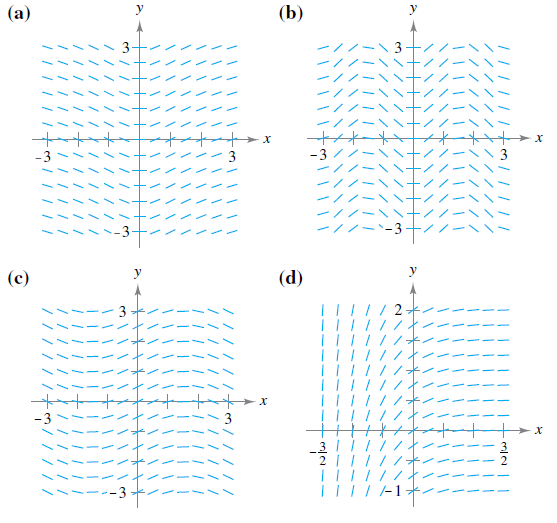Chapter 6.1, Problem 58E

Chapter
Section
Textbook Problem

Matching In Exercises 57–60, match the differential equation with its slope field. [The slope fields are labeled (a), (b), (c), and (d).]d y d x = 1 2 cos x

To determine
The differential equation associated with its given slope field.Explanation

Given: dydx=12cosx and the four graphs of the slope field.

a). b).

c). d).

Explanation:

As dydx=12cosx, it implies that the slope is oscillatory in nature.

We start by creating a table showing the slopes at several points.

The table which is shown below is a smallsample. The slopes at many other points should be calculated to get a representative slope field

Still sussing out bartleby?

Check out a sample textbook solution.

See a sample solution

The Solution to Your Study Problems

Bartleby provides explanations to thousands of textbook problems written by our experts, many with advanced degrees!

Get Started

Evaluate the integral. excosxdx

Calculus (MindTap Course List)

In Problems 35-42, simplify each complex fraction. 37.

Mathematical Applications for the Management, Life, and Social Sciences

Evaluate the integral, if it exists. 02y21+y3dy

Single Variable Calculus: Early Transcendentals